## Chromatic Number-

Before you go through this article, make sure that you have gone through the previous article on Chromatic Number.

We gave discussed-

• Graph Coloring is a process of assigning colors to the vertices of a graph.
• It ensures that no two adjacent vertices of the graph are colored with the same color.
• Chromatic Number is the minimum number of colors required to properly color any graph.

In this article, we will discuss how to find Chromatic Number of any graph.

## Graph Coloring Algorithm-

• There exists no efficient algorithm for coloring a graph with minimum number of colors.
• Graph Coloring is a NP complete problem.

However, a following greedy algorithm is known for finding the chromatic number of any given graph.

## Greedy Algorithm-

### Step-01:

Color first vertex with the first color.

### Step-02:

Now, consider the remaining (V-1) vertices one by one and do the following-

• Color the currently picked vertex with the lowest numbered color if it has not been used to color any of its adjacent vertices.
• If it has been used, then choose the next least numbered color.
• If all the previously used colors have been used, then assign a new color to the currently picked vertex.

## Drawbacks of Greedy Algorithm-

There are following drawbacks of the above Greedy Algorithm-

• The above algorithm does not always use minimum number of colors.
• The number of colors used sometimes depend on the order in which the vertices are processed.

Also Read- Types of Graphs in Graph Theory

## Problem-01:

Find chromatic number of the following graph-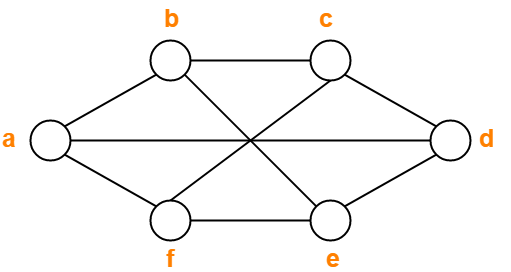## Solution-

Applying Greedy Algorithm, we have-

 Vertex a b c d e f Color C1 C2 C1 C2 C1 C2

From here,

• Minimum number of colors used to color the given graph are 2.
• Therefore, Chromatic Number of the given graph = 2.

The given graph may be properly colored using 2 colors as shown below-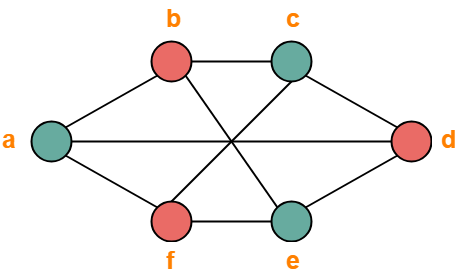## Problem-02:

Find chromatic number of the following graph-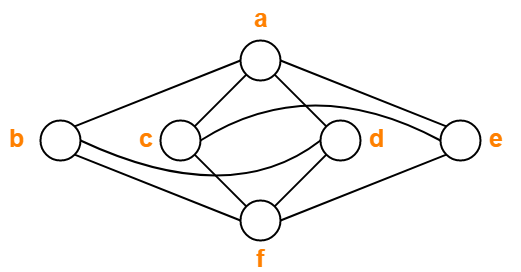## Solution-

Applying Greedy Algorithm, we have-

 Vertex a b c d e f Color C1 C2 C2 C3 C3 C1

From here,

• Minimum number of colors used to color the given graph are 3.
• Therefore, Chromatic Number of the given graph = 3.

The given graph may be properly colored using 3 colors as shown below-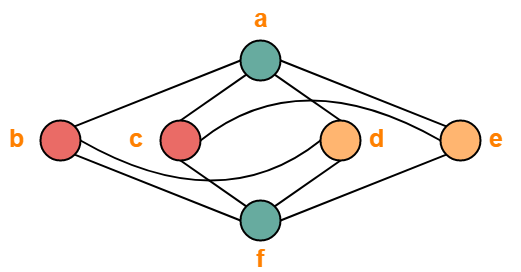## Problem-03:

Find chromatic number of the following graph-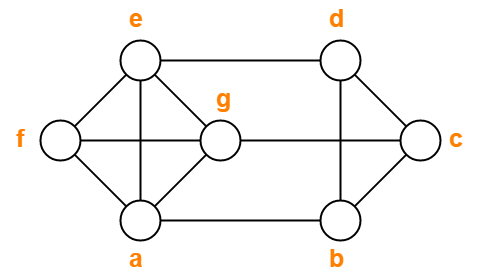## Solution-

Applying Greedy Algorithm, we have-

 Vertex a b c d e f g Color C1 C2 C1 C3 C2 C3 C4

From here,

• Minimum number of colors used to color the given graph are 4.
• Therefore, Chromatic Number of the given graph = 4.

The given graph may be properly colored using 4 colors as shown below-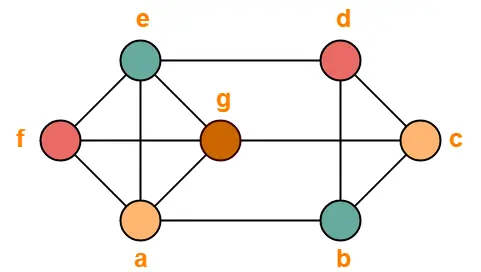## Problem-04:

Find chromatic number of the following graph-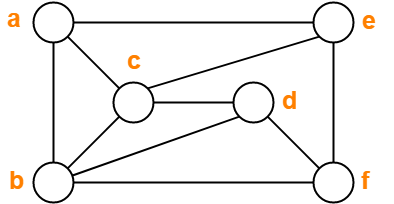## Solution-

Applying Greedy Algorithm, we have-

 Vertex a b c d e f Color C1 C2 C3 C1 C2 C3

From here,

• Minimum number of colors used to color the given graph are 3.
• Therefore, Chromatic Number of the given graph = 3.

The given graph may be properly colored using 3 colors as shown below-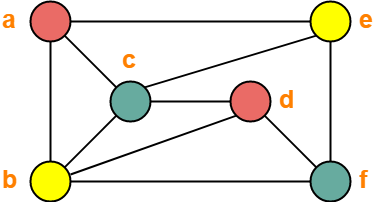## Problem-05:

Find chromatic number of the following graph-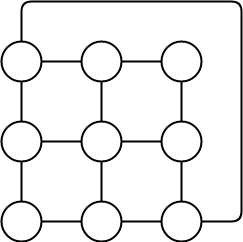## Solution-

Applying Greedy Algorithm,

• Minimum number of colors required to color the given graph are 3.
• Therefore, Chromatic Number of the given graph = 3.

The given graph may be properly colored using 3 colors as shown below-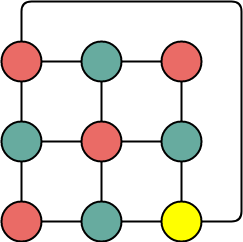To gain better understanding about How to Find Chromatic Number,

Watch this Video Lecture

Get more notes and other study material of Graph Theory.

Watch video lectures by visiting our YouTube channel LearnVidFun.

## Graph Coloring-

 Graph Coloring is a process of assigning colors to the vertices of a graph such that no two adjacent vertices of it are assigned the same color.

• Graph Coloring is also called as Vertex Coloring.
• It ensures that there exists no edge in the graph whose end vertices are colored with the same color.
• Such a graph is called as a Properly colored graph.

### Graph Coloring Example-

The following graph is an example of a properly colored graph-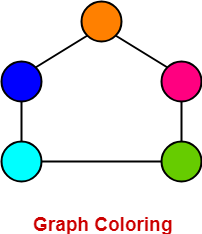In this graph,

• No two adjacent vertices are colored with the same color.
• Therefore, it is a properly colored graph.

### Graph Coloring Applications-

Some important applications of graph coloring are as follows-

• Map Coloring
• Preparing Time Table
• Assignment
• Conflict Resolution
• Sudoku

## Chromatic Number-

 Chromatic Number is the minimum number of colors required to properly color any graph. OR Chromatic Number is the minimum number of colors required to color any graph such that no two adjacent vertices of it are assigned the same color.

### Chromatic Number Example-

Consider the following graph-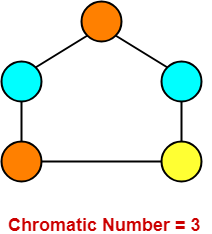In this graph,

• No two adjacent vertices are colored with the same color.
• Minimum number of colors required to properly color the vertices = 3.
• Therefore, Chromatic number of this graph = 3.
• We can not properly color this graph with less than 3 colors.

Also Read- Types of Graphs in Graph Theory

## Chromatic Number Of Graphs-

Chromatic Number of some common types of graphs are as follows-

## 1. Cycle Graph-

• A simple graph of ‘n’ vertices (n>=3) and ‘n’ edges forming a cycle of length ‘n’ is called as a cycle graph.
• In a cycle graph, all the vertices are of degree 2.

### Chromatic Number

• If number of vertices in cycle graph is even, then its chromatic number = 2.
• If number of vertices in cycle graph is odd, then its chromatic number = 3.

### Examples-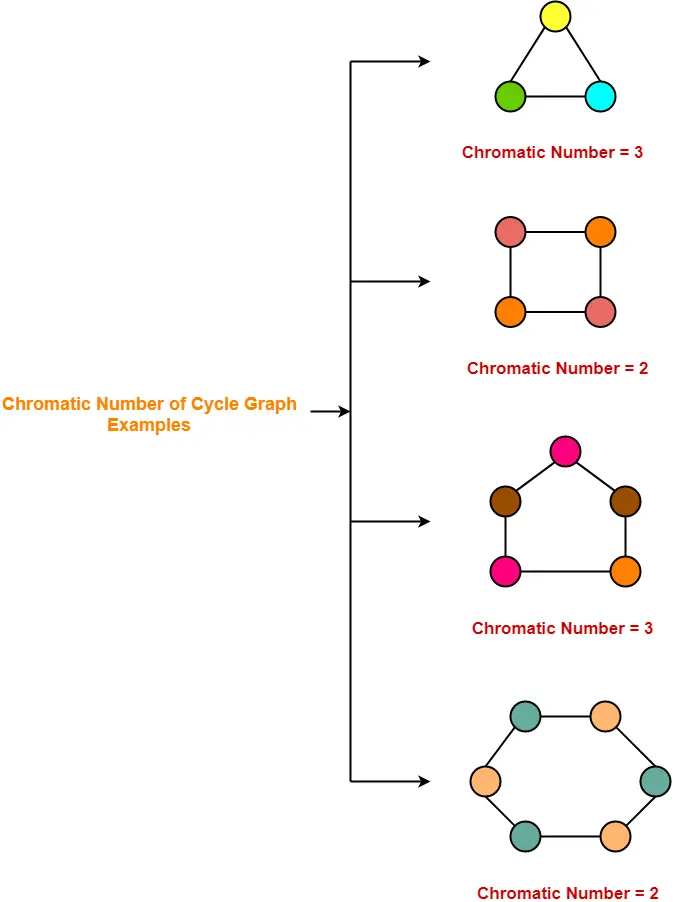## 2. Planar Graphs-

A Planar Graph is a graph that can be drawn in a plane such that none of its edges cross each other.

### Chromatic Number

Chromatic Number of any Planar Graph

= Less than or equal to 4

### Examples-

• All the above cycle graphs are also planar graphs.
• Chromatic number of each graph is less than or equal to 4.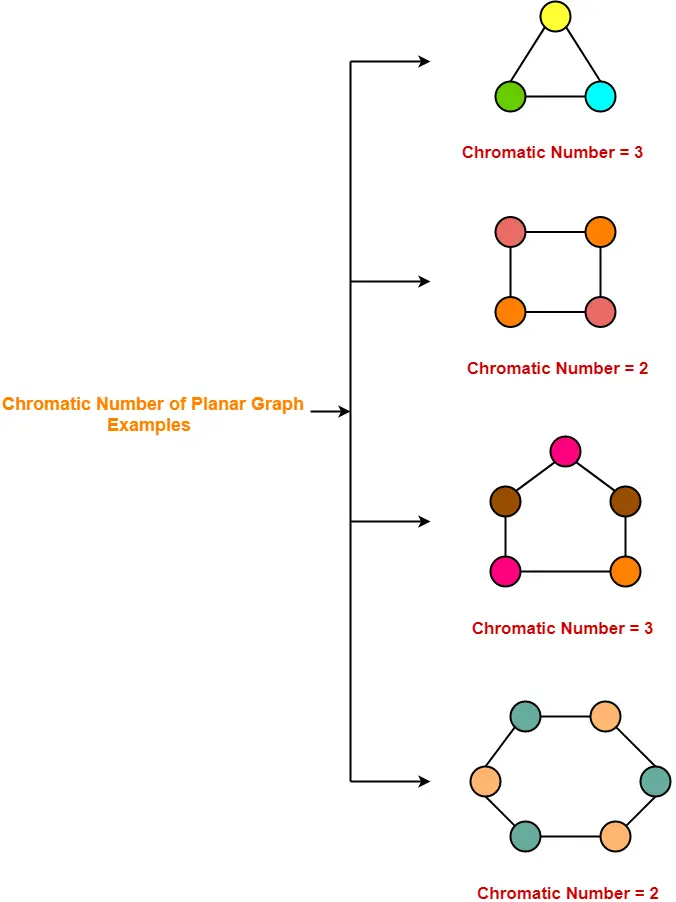## 3. Complete Graphs-

• A complete graph is a graph in which every two distinct vertices are joined by exactly one edge.
• In a complete graph, each vertex is connected with every other vertex.
• So to properly it, as many different colors are needed as there are number of vertices in the given graph.

### Chromatic Number

Chromatic Number of any Complete Graph

= Number of vertices in that Complete Graph

### Examples-## 4. Bipartite Graphs-

• A Bipartite Graph consists of two sets of vertices X and Y.
• The edges only join vertices in X to vertices in Y, not vertices within a set.

### Chromatic Number

Chromatic Number of any Bipartite Graph

= 2

### Example-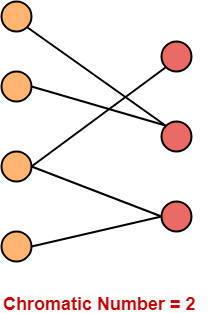## 5. Trees-

• A Tree is a special type of connected graph in which there are no circuits.
• Every tree is a bipartite graph.
• So, chromatic number of a tree with any number of vertices = 2.

### Chromatic Number

Chromatic Number of any tree

= 2

### Examples-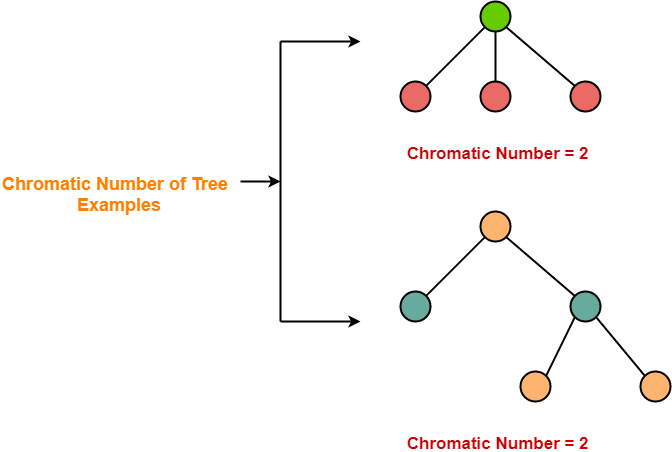To gain better understanding about Graph Coloring & Chromatic Number,

Watch this Video Lecture

Next Article- Graph Coloring Algorithm

Get more notes and other study material of Graph Theory.

Watch video lectures by visiting our YouTube channel LearnVidFun.